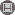دوره 7، شماره 2 - ( 8-1391 )                   جلد 7 شماره 2 صفحات 83-91 | برگشت به فهرست نسخه ها

BibTeX | RIS | EndNote | Medlars | ProCite | Reference Manager | RefWorks
Send citation to:Ashrafi N, Ahmadi Z. WEAKLY g(x)-CLEAN RINGS. IJMSI. 2012; 7 (2) :83-91
URL: http://ijmsi.ir/article-1-353-fa.html
WEAKLY g(x)-CLEAN RINGS. مجله علوم ریاضی و انفورماتیک. 1391; 7 (2) :83-91

چکیده:

A ring \$R\$ with identity is called ``clean'' if \$~\$for every element \$ain R\$, there exist an idempotent \$e\$ and a unit \$u\$ in \$R\$ such that \$a=u+e\$. Let \$C(R)\$ denote the center of a ring \$R\$ and \$g(x)\$ be a polynomial in \$C(R)[x]\$. An element \$rin R\$ is called ``g(x)-clean'' if \$r=u+s\$ where \$g(s)=0\$ and \$u\$ is a unit of \$R\$ and, \$R\$ is \$g(x)\$-clean if every element is \$g(x)\$-clean. In this paper we define a ring to be weakly \$g(x)\$-clean if each element of \$R\$ can be written as either the sum or difference of a unit and a root of \$g(x)\$.

نوع مطالعه: پژوهشي | موضوع مقاله: عمومى

ارسال نظر درباره این مقاله : نام کاربری یا پست الکترونیک شما:کلیه حقوق این وب سایت متعلق به نشریه علوم ریاضی و انفورماتیک می باشد.

طراحی و برنامه نویسی : یکتاوب افزار شرق